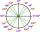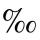# Lift

The largest angle at which the lift rises is 16°31'. Give climb angle in permille.

Result

p =  296.53

#### Solution:Leave us a comment of example and its solution (i.e. if it is still somewhat unclear...):Be the first to comment!## Next similar examples:

1. ClimbFor horizontal distance 4.2 km road rise by 6.3 m. Calculate the road pitch in ‰ (permille, parts per thousand).
2. CosineThe point (8, 6) is on the terminal side of angle θ. cos θ = ?
3. Reference angleFind the reference angle of each angle:What is greater angle? -0.7 radians or -0.7π radians?
5. PermillHow many permill is 978 from 84370?
6. Theorem proveWe want to prove the sentence: If the natural number n is divisible by six, then n is divisible by three. From what assumption we started?
7. Linear systemSolve a set of two equations of two unknowns: 1.5x+1.2y=0.6 0.8x-0.2y=2
8. Two equationsSolve equations (use adding and subtracting of linear equations): -4x+11y=5 6x-11y=-5
9. One halfOne half of ? is: ?
10. 6 termsFind the first six terms of the sequence. a1 = 7, an = an-1 + 6
11. PowersExpress the expression ? as the n-th power of the base 10.
12. Volleyball8 girls wants to play volleyball against boys. On the field at one time can be six players per team. How many initial teams of this girls may trainer to choose?
13. First manWhat is the likelihood of a random event where are five men and seven women first will leave the man?
14. TreesA certain species of tree grows an average of 0.5 cm per week. Write an equation for the sequence that represents the weekly height of this tree in centimeters if the measurements begin when the tree is 200 centimeters tall.
15. Functions f,gFind g(1) if g(x) = 3x - x2 Find f(5) if f(x) = x + 1/2
16. Factory and divisionsThe factory consists of three auxiliary divisions total 2,406 employees. The second division has 76 employees less than 1st division and 3rd division has 212 employees more than the 2nd. How many employees has each division?
17. Nineteenth memberFind the nineteenth member of the arithmetic sequence: a1=33 d=5 find a19